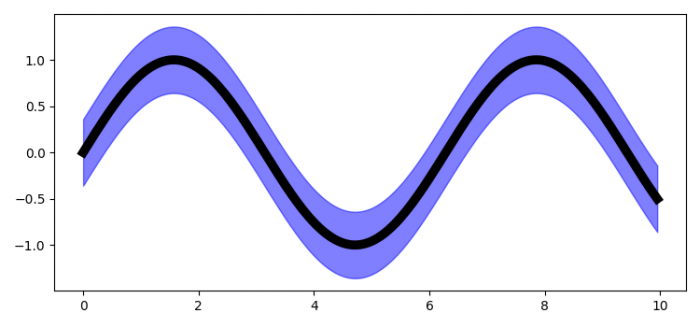# How to visualize 95% confidence interval in Matplotlib?

To visualize 95% confidence interval in Matplotlib, we can take the following steps −

• Set the figure size and adjust the padding between and around the subplots.

• Create x and y data sets.

• Get the confidence interval dataset.

• Plot the x and y data points using plot() method.

• Fill the area within the confidence interval range.

• To display the figure, use show() method.

## Example

from matplotlib import pyplot as plt
import numpy as np

plt.rcParams["figure.figsize"] = [7.50, 3.50]
plt.rcParams["figure.autolayout"] = True

x = np.arange(0, 10, 0.05)
y = np.sin(x)

# Define the confidence interval
ci = 0.1 * np.std(y) / np.mean(y)

plt.plot(x, y, color='black', lw=7)

plt.fill_between(x, (y-ci), (y+ci), color='blue', alpha=0.5)

plt.show()

## Output